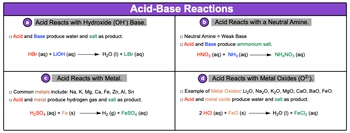Start typing, then use the up and down arrows to select an option from the list.## GOB Chemistry

Learn the toughest concepts covered in your GOB - General, Organic, and Biological Chemistry class with step-by-step video tutorials and practice problems.

Acids and Bases

# Acid-Base Reactions

Acids can react with several different compounds such as hydroxide bases, amines, metals and metal oxides.

Common Acid-Base Reactions
1
concept

## Acid-Base Reactions Concept 13m
Play a video:
common acid base reactions include reactions of acids with a hydroxide basis. Always minus be neutral means see metals and D. Metal oxides. Now an amine is just a compound that contains nitrogen and hydrogen or carbon, nitrogen and hydrogen. And then remember that neutral means are weak bases. So if you take a look here at our different types of acid base reactions and the first one we have acids reacting with the hydroxide base. We're gonna stay here. An acid and a base will produce water and a salt as a product. This salt is just an ionic compound. So here we have an example of an acid base reaction here would say that the H plus from the acid combined with the O. H minus from the base to give us water as a liquid. And an L. I. Positive and br minus will combine together to give me my salt. So, lbr acquis here we have acids reacting with a neutral amine. So neutral means remember our week basis An example. We have NH three which is ammonia. It's composed of nitrogen and hydrogen. And here we have what's called methyl amine. It has carbon, nitrogen and hydrogen involved. Here we're going to say that this acid and base together produce a salt. So here we have nitric acid reacting with ammonia. What's going to happen is you're gonna create ammonium nitrate as a salt. Remember this is an ionic compound Here we can have asses reacting with metals. So the common metals can include sodium, potassium, magnesium, calcium, iron, zinc, aluminum and tin. Here we're going to say that our acid and metal will produce hydrogen gas. So H2 gas and will also produce assault. Yeah. Alright, so here we have sulfuric acid reacting with a metal, We're gonna produce H2 gas and then the iron will combine with the sulfate to give me iron to sulphate Equus. Alright then finally we can have an acid reacting with a metal oxide. So examples of metal oxides, we can see lithium oxide, sodium oxide, potassium, magnesium, calcium, barium, and iron to oxide. Here we're gonna say the acid and the metal oxide. They're going to produce water as a as a product as well as again a salt. So here we'd say that we're going to create water from these reacting and then we also create iron to chloride. Here, we're not really worrying about balancing. We're just looking to see what kind of products were making from each of these. Remember these are pattern recognition types of examples. You have to understand what kind of compound is the acid reacting with to help you to determine the products that will form.2
example

## Acid-Base Reactions Example 152s
Play a video:
here it says, complete the following reaction. Here we have an acid in the form of Hydrofluoric acid reacting with a hydroxide basis in the form of potassium hydroxide. So what's going to happen treat this like a regular double displacement reaction. We had sulfuric um hydro for gas, it which is composed of age plus and F minus. And then we have potassium hydroxide which is K plus an O. H minus. Remember opposites attract this positive and this negative will now attract to give me water as a liquid. And then this positive in this negative will combine to give me my salt KF or potassium fluoride, Aquarius. So this would represent the two products that are formed within this reaction. Here. Everything is already balanced. It would be a coefficient of one for each of the compounds within this equation.
3
Problem

Write a balanced chemical equation for the following acid-base reaction:
Bromic acid reacting with hydrazine (N2H4).

4
Problem

Write a balanced chemical equation, include phases, for the following acid reaction:HClO3 reacting with Zn metal.

5
Problem

Write a balanced chemical equation, include phases, for the following acid reaction:HBr reacting with Li2O.